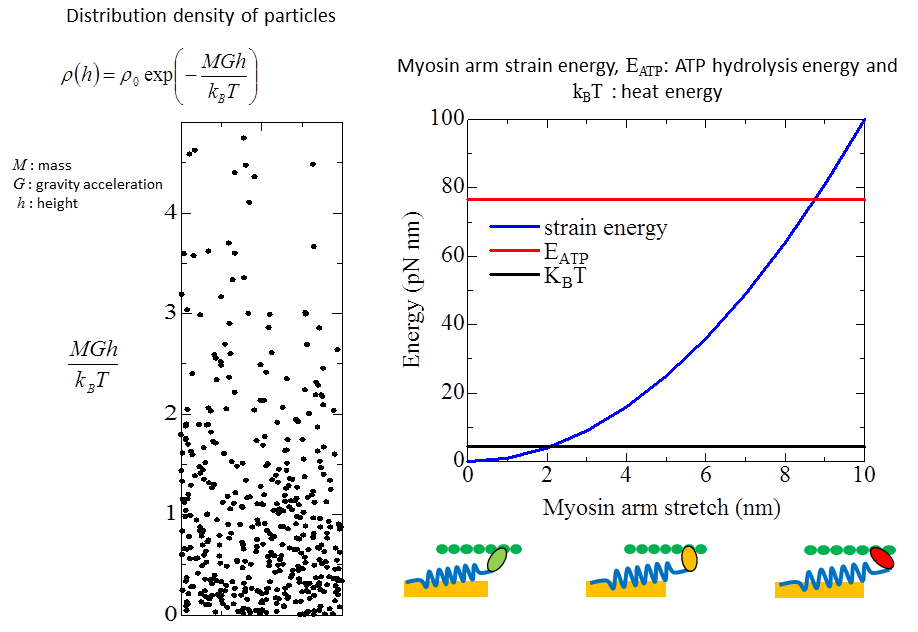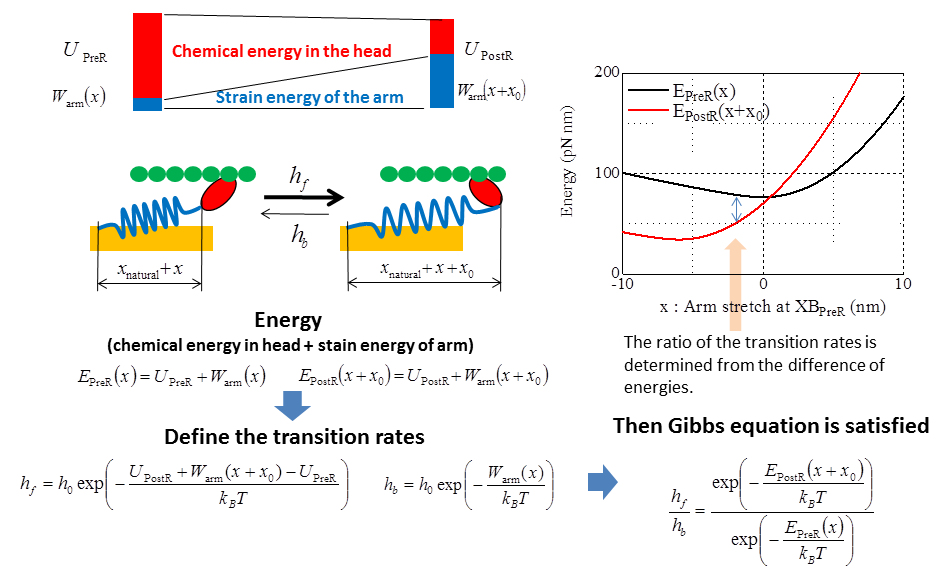## Energy aware transition control

How are the transformations between the chemical energy of ATP hydrolysis in the myosin head and the mechanical energy given by the myosin arm strain performed? At the moment, we do not have enough knowledge to model precisely this process. What we can do with our best knowledge may be to make the transitions as the state distribution converges to the statistical equilibrium.

### Boltzmann factor

Particles with energies of a similar order of magnitude to the heat energy KBT present stochastic behavior. As an example, the density distribution of particles in the atmosphere is shown in the left figure. It follows the Boltzmann distribution. The energy generated by the myosin head rotation or the ATP hydrolysis energy is of a similar order of magnitude to the heat energy KBT as depicted in the right figure. Thus the state and arm stretch distributions of myosin molecules in equilibrium should be also stochastically determined according to the Boltzmann distribution.### Transition rate for a head rotation

Here an approach to determine the head rotation transition rates to meet such a request is shown. In this approach, we assume that one particle is composed of a myosin head and an arm attached to it. We assume that the energy of a particle is given by the sum of chemical energy stored in its head and the strain energy of its arm. With this situation, we determine the transition rates so that the state density of the particles obeys the Boltzmann distribution in equilibrium. Then the Gibbs equation must be fulfilled with the transition rates. This consideration leads us to define the transition rates as shown below where we only have to determine a parameter h0 that determines the speed of transitions. In the figures below, U_PreR and U_PostR are chemical energies stored in the myosin head in the states XB_PreR and XB_PostR respectively. xnatural is the natural length of myosin arm, and x0 is the stroke distance given at the transition from XB_PreR and XB_PostR. Here the difference of chemical energies U_PreR - U_PostR is consumed by a head rotation to produce a strain increment of x0. The ratio of transition rates is determined by the ratio of Boltzmann factors of the two states in the Gibbs equation. The definition of the transition rates hf and hb is just an example that fulfills the Gibbs equation.